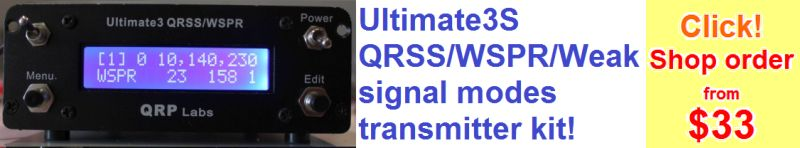This is the 26'th test flight of the new U4B tracker. For details see below.

Callsign is VE3KCL, WSPR transmission on 20m, is minute :04 and telemetry in minute :06 and telemetry call 0x4xxx

## Transmitter

This is the 26'th test flight of the new U4B tracker. U4B-26 was assembled by Dave VE3KCL. This YouTube video explains the features of the U4B tracker: https://youtu.be/CqNZeh575xw

U4B Basic program listing

```/
Name           Type   Size  Blocks  Start  Compiled
..
MAIN           TEXT    282       3      2       Yes
BA             TEXT    100       1      1       Yes
CLS            TEXT    137       2     13       Yes
ETP            TEXT     26       1      7       Yes
GPST           TEXT    155       2      8       Yes
SPT            TEXT     84       1      5       Yes
TU             TEXT     34       1     10       Yes
TV             TEXT     41       1     11       Yes
..

MAIN

5 LET C = 83
6 LET R = 0
7 LET HP = 0
12 LET FR = 26999972
21 LET R = R + 1
IF R > 3
LET HP = 1
22 GOTO 25
23 ENDIF
24 LET HP = 0
25 LET CE = 0
RUN "GPST"
RUN "SPT"
RUN "ETP"
RUN "CLS"
RUN "TU"
RUN "TV"
RUN "PT"
OUT 19 0
SLEEP 10 3
RUN "BA"
IF HP = 1
CW 0 14096932 1 0 "T"
GOTO 44
ENDIF
CW 0 14096890 1 0 "T"
44 TELE U V
50 GOTO 12

BA   /BALLOON GLYPH

CW 0 14096900 5 0 "*01IFLCNAP8Q7R6R5S0S0S5R6R7Q*"
CW 0 14096912 5 0 "*01555 902906800  6380490402*"

CLS  / CLOUD SENSOR

LET D = INA 3
LET Q = 70
LET M = 500
FOR T = 1 TO Q
LET D = D + INA 3
NEXT
LET D = D / M
IF D > 50
LET D = D / 10 + 50
ENDIF
IF D = 0
LET D = 1
ENDIF
IF D > 99
LET D = 99
ENDIF
PRINT "D", D

ETP    /EXTERNAL TEMP SENSOR NOTE ADD 2 TO THE ADDRESS SO     I2CR 146 0  WITH A0 HIGH

LET A = I2CR 146 0
PRINT "A", A

GPST       /GPS AND GPS TIME TO LOCK

LET G = VAL "#3.2TM"
LET G = 60 * G + VAL "#5.2TM"
GPS 290 "\$PSIMNAV,W,3*3A"
LET H = VAL "#3.2TM"
LET H = 60 * H + VAL "#5.2TM"
LET I = H - G
IF I > 320
LET I = 320
ENDIF
PRINT "I", I
OUT 19 GL
LET FR = 26999972

SPT       / SOLAR POWER TEST

LET E = 0
OUT 7 1
FOR B = 1 TO 8
LET E = E + BT
NEXT
LET E = E / 8
PRINT "E", E
OUT 7 0

TU        / TELE U

LET U = E * 100 + A + 50
PRINT "U", U

TV      / TELE V

LET V = HP * 100000 + I * 100 + D
PRINT "V", V```
` `

TX Photos

Launch Photos